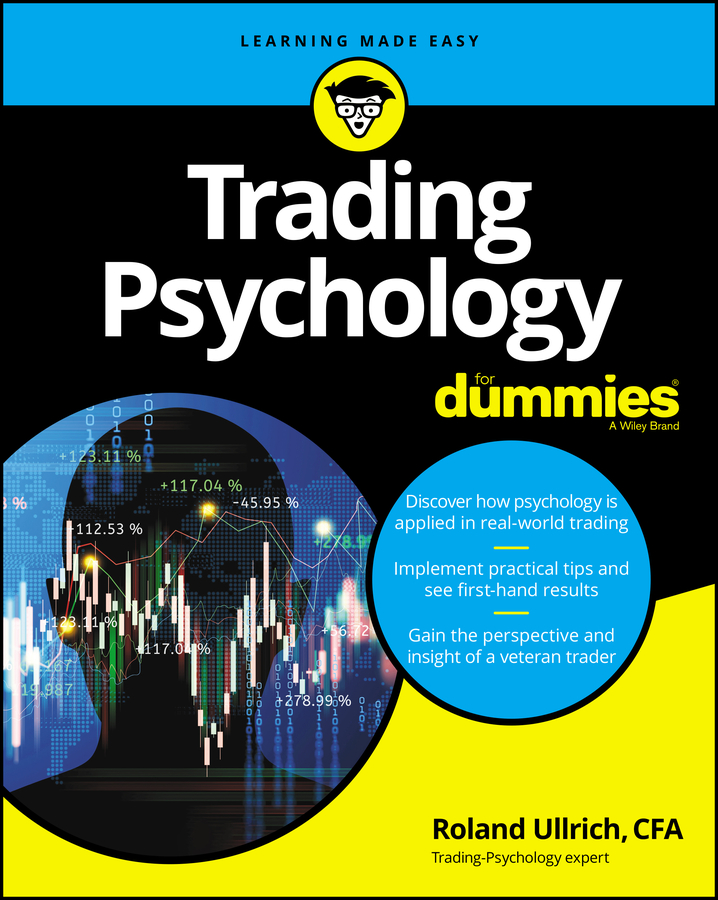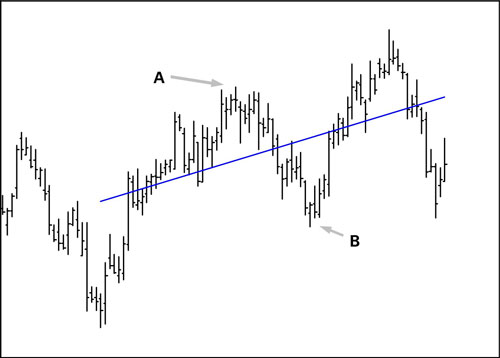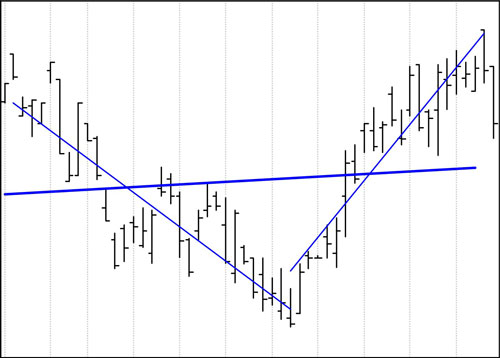##### Trading Psychology For DummiesOn a trading chart, you can draw a line (called the linear regression line) that goes through the center of the price series, which you can analyze to identify trends in price. Although you can’t technically draw a straight line through the center of each trading chart price bar, the linear regression line minimizes the distance from itself to each price close along the line and thus provides a way to evaluate trends.

In this figure, the linear regression line doesn’t actually go through the center of each price bar. In fact, some price bars are quite far away from the line. But if you look more closely, you can see that no other line gets you as close to Point A and to Point B at the same time. Only one linear regression exists for any set of prices on the chart.Despite its somewhat intimidating name, the linear regression should have you breathing a sigh of relief right now because nothing is subjective or judgmental about it. It’s “science.” Everybody gets the identical line if they are given the same chart and the same starting and ending points:

• Pros: A linear regression is the true, pure trendline. If you accept the core concept of technical analysis, that a trend will continue in the same direction, at least for a while, then you can extend the true trendline and obtain a forecast.

In some software packages, a linear regression extension is called exactly that — a time-series forecast. This tool is tremendously useful. You have created a high-probability forecast for the upcoming period that gives you perspective on what to expect.

• Cons: The bad news is that the linear regression line can slope this way or that way or no way (horizontal), depending on where you start and stop drawing. If you take a V-shaped price series like the one in this figure and draw a single linear regression line, you get . . . garbage.Unless you have a very long holding period in mind, this chart shows two trends, and you need two linear regressions to reflect that. This conundrum leads to the common-sense observation that the less daylight between the line and the price points on the chart, the better the fit and the more likely it is that extending the line is a valid technique.